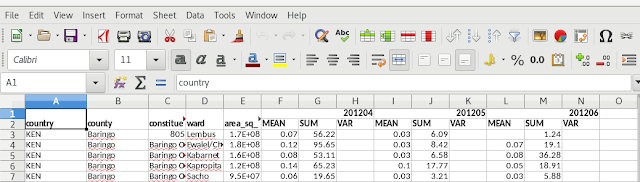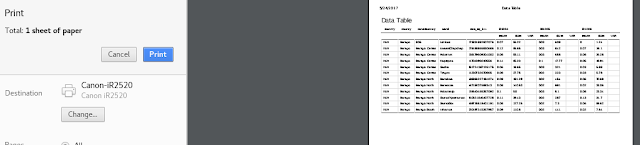This is a writeup on some code (read hack) that we had to put together quickly to solve a problem. That is exporting data with multiple headers and merged rows/columns in the headers from an R Shiny datatable. Shiny datatables (Shiny/DT) which is based on (datatables.net) is a fantastic way to quickly display tabular data in an R Shiny web application and to export it to multiple formats. The changes required to try out the code are posted on the shiny/DT github issues page (here) and we thought we would just publish here for anyone who might want to give it a go and maybe even expand on it.

Hi all,
This is an extension of lisarush 's and dfader 's code over at (https://datatables.net/forums/discussion/40854/how-to-add-second-footer-to-print-in-tfoot?) to get multiple headers to work in csv, xlsx, pdf and print.
I also have added a basic merge working for colspan and rowspan headers in xlsx. It does not work in firefox but does in chrome and opera browsers and I don't know why yet. Hopefully someone can help with that. It should be possible to port this (at least the logic) for the footer and body as well.
I am not sure if the rstudio/DT code is modified from the datatables.net code but I found some differences and the edit suggestions at the datatables.net forums had to be altered to work. So these changes should work directly in rstudio/DT and with modification at datatables.net. Anyway, hopefully, this will help somebody.
Disclaimer: I am not a javascript programmer and so this code is not tested, might have bugs and might affect the currently functioning DT code. Use at your own risk and feel free to edit the code as you see fit. Any questions, comments or critique are welcome.

### 1.In "dataTables.buttons.min.js" file

(You will need to deminify the files first)
a. Add this function: Insert in the section with `m.background = function(...` and similar code
``````    /* ----- BEGIN added Code ----- */
var numRows = thRows.length;
var matrix = [];

// Iterate over each row of the header and add information to matrix.
for ( var rowIdx = 0;  rowIdx < numRows;  rowIdx++ ) {
var \$row = \$(thRows[rowIdx]);

// Iterate over actual columns specified in this row.
var \$ths = \$row.children("th");
for ( var colIdx = 0;  colIdx < \$ths.length;  colIdx++ )
{
var \$th = \$(\$ths.get(colIdx));
var colspan = \$th.attr("colspan") || 1;
var rowspan = \$th.attr("rowspan") || 1;
var colCount = 0;

// ----- add this cell's title to the matrix
if (matrix[rowIdx] === undefined) {
matrix[rowIdx] = [];  // create array for this row
}
// find 1st empty cell
for ( var j = 0;  j < (matrix[rowIdx]).length;  j++, colCount++ ) {
if ( matrix[rowIdx][j] === "PLACEHOLDER" ) {
break;
}
}
var myColCount = colCount;
matrix[rowIdx][colCount++] = \$th.text();

// ----- If title cell has colspan, add empty titles for extra cell width.
for ( var j = 1;  j < colspan;  j++ ) {
matrix[rowIdx][colCount++] = "";
}

// ----- If title cell has rowspan, add empty titles for extra cell height.
for ( var i = 1;  i < rowspan;  i++ ) {
var thisRow = rowIdx+i;
if ( matrix[thisRow] === undefined ) {
matrix[thisRow] = [];
}
// First add placeholder text for any previous columns.
for ( var j = (matrix[thisRow]).length;  j < myColCount;  j++ ) {
matrix[thisRow][j] = "PLACEHOLDER";
}
for ( var j = 0;  j < colspan;  j++ ) {  // and empty for my columns
matrix[thisRow][myColCount+j] = "";
}
}
}
}
return matrix;
};
``````
b. Replace this code:
``````a = b.columns(c.columns).indexes().map(function(a) {
}).toArray()
``````
with this code:
``````/*BEGIN ADD*/ headerMatrix = getHeaders( b.settings() ) /*END ADD*/
``````
c. Replace this code:
``````f = a.length
``````
with this code:
``````f = headerMatrix[headerMatrix.length - 1].length
``````
d. Then replace:
``````return {
footer: g,
body: j
}
``````
with:
``````return {
footer: g,
body: j
}
``````

### 2.In "buttons.html5.min.js" file

a. Add multiple headers in xlsx i. Find the section s.ext.buttons.excelHtml5 and the action: function ii. Replace this code:
``````if(c.header){d(b.header,e);g("row c",f).attr("s","2")}
``````
with this code:
``````for (i = 0; i < b.header.length; i++){
g("row c", f).attr("s", "2"); //maybe should be outside the loop?
}
``````
b. Add multiple headers in pdf i. Find the section s.ext.buttons.pdfHtml5 and the action: function ii. Replace this code:
``````c.header&&b.push(g.map(a.header,function(a){return{text:typeof a==="string"?a:a+"",style:"tableHeader"}}));
``````
with
``````if(c.header) /*CHANGE*/
for(i = 0; i < a.header.length; i++)
return {
text: typeof a === "string" ? a : a + "",
}
}));
``````

### 3.In "buttons.print.min.js"

c. Add multiple headers in print i. Find the action: function ii. Replace this code:
``````f.header&&(b += "<thead>" + a(c.header[i], "th") + "</thead>");
``````
with
``````if(f.header)
for(i = 0; i < c.header.length; i++)
``````

## B. Merge colspan/rowspan headers in xlsx

(Note: This will only work if you have gone through the earlier steps in part A)

### 1.Edit the "buttons.html5.min.js" file

a. Back in the s.ext.buttons.excelHtml5 section b. Replace the code we added earlier for multiple headers:
``````for (i = 0; i < b.header.length; i++){
g("row c", f).attr("s", "2"); //maybe should be outside the loop?
}
``````
with this code:
``````            if (c.header) {
{
//for each column (cell) in the row
{
//look for a non-colspan/rowspan cell
{
var startRow = i;
var startCol = j;
var endRow = i;
var endCol = j;

if(b.header[i][j+1] == "") //is the cell to the right a colspan?
{
startCol = j;
endCol = j+1;

//get to the last column in the colspan
{
//b.header[i][endCol] = ""; //Use if cspan is a special char/sequence
endCol++;
}
endCol--;
}

if(b.header[i+1][j] == "") //is the cell below a rowspan?
{
startRow = i;
endRow = i+1;

//get to the last row in the rowspan
{
//b.header[endRow][j] = ""; //Use if rowspan is a special char/sequence
endRow++;
}
}

//create and store merge ranges
//if endCol or endRow show movement
if(startRow != endRow || startCol != endCol)
{
sC = colLetter(startCol); //convert startCol to excel column letter
sR = startRow+1;
eC = colLetter(endCol); //conver endCol to excel column letter
eR = endRow;

//console.log("sC="+sC);
merges[mgCnt] = sC+""+sR; //start of range

//console.log("endrow > startrow="+endRow+">"+startRow);
//console.log("endCol > startcol="+endCol+">"+startCol);

if(endCol > startCol) //end column
merges[mgCnt] = merges[mgCnt] + ":" + eC;
else
merges[mgCnt] = merges[mgCnt] + ":" + sC;

if(endRow > startRow) //end row
merges[mgCnt] = merges[mgCnt] + eR;
else
merges[mgCnt] = merges[mgCnt] + sR;

//console.log("merges[mgCnt]="+merges[mgCnt]);

mgCnt++; //increment number of merge ranges
}
}
}
}

for (i = 0; i < b.header.length; i++){
g("row c", f).attr("s", "2"); //maybe should be outside the loop?
}
}
``````
c. In between this code:
``````            for (var i = 0, l = b.body.length; i < l; i++) d(b.body[i], e);
if (c.footer && b.footer) {
d(b.footer, e);
g("row:last c", f).attr("s", "2")
}
``````
and this code:
``````d = o(f, "cols");
``````
``````            //if we have merges
if (mgCnt > 0)
{
//create a mergeCells section
z = o(f, "mergeCells", {
attr: {
count: mgCnt,
}
});

//add each merge range as a child
for(i=0;i<mgCnt;i++)
{
n = o(f, "mergeCell", {
attr: {
ref: merges[i]
}
});
z.appendChild(n);
}
}
if(z.children.length > 0)
g("worksheet", f).append(z) //add to the worksheet
``````
That's it. You're done! Some screenshots:The datatable in ShinyThe datatable exported to .xlsxThe datatable exported to .pdfThe datatable print preview

1.\$('#listpersonal').dataTable({
retrieve: true,
"ajax" : {
"url" : "/HRMS/getPersonlDetails?division="+division+"&&project="+project,
"dataSrc" : "dataBean",
"type" : "GET",

},
"columns" : [

{
data : "personalparentid"
}, {
data : "emailid"
}, {
data : "maritalstatus"
}, {
data : "mobilenumber"
}, {
data : "alternatemobilenumber"
}, {
data : "drivinglicenceno"
}, {
data : "drivinglicencevalidity"
}, {
data : "passportnumber"
}, {
data : "passportvalidity"
}, {
}, {
data : "pancardnumber"
}, {
data : "bankname"
}, {
data : "bankbranch"
}, {
data : "branchifsccode"
}, {
data : "accountnumber"
}, {
data : "cardnumber"
}, {
}, {
}, {
data : "bloodgroup"
}, {
data : "doBirth"
},
],
"scrollX": true,
"scrollY": "400px",
"scrollCollapse": true,
"paging": false,
dom: 'Bfrtip',

buttons : [
{
extend : 'print'
, action : function( e, dt, button, config ) {
dt_print( e, dt, button, config, true )
} /* true here means table has child rows */
}
]
});
\$('#personalListModal').modal('show');

2.